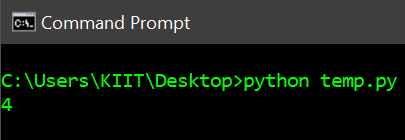GeeksforGeeks App
Open AppBrowser
Continue

# Count the number of times a letter appears in a text file in Python

In this article, we will be learning different approaches to count the number of times a letter appears in a text file in Python. Below is the content of the text file gfg.txt that we are going to use in the below programs:Now we will discuss various approaches to get the frequency of a letter in a text file.

Method 1: Using the in-built count() method.

Approach:

• Store the content of the file in a variable.
• Use the count() method with the argument as a letter whose frequency is required.
• Display the count of the letter.

Implementation:

## Python3

 `# Program to get letter count in a text file` `# explicit function to return the letter count``def` `letterFrequency(fileName, letter):``    ``# open file in read mode``    ``file` `=` `open``(fileName, ``'r'``)` `    ``# store content of the file in a variable``    ``text ``=` `file``.read()` `    ``# using count()``    ``return` `text.count(letter)`  `# call the function and display the letter count``print``(letterFrequency(``'gfg.txt'``, ``'g'``))`

Output:Method 2: Iterate through the file content in order to compare each character with the given letter.

Approach:

• Store the content of the file in a variable.
• Assign a counter count variable with 0.
• Iterate through each character, if the character is found to be the given letter then increment the counter.
• Display the count of the letter.

Implementation:

## Python3

 `# Program to get letter count in a text file` `# explicit function to return the letter count``def` `letterFrequency(fileName, letter):``    ``# open file in read mode``    ``file` `=` `open``(fileName, ``"r"``)` `    ``# store content of the file in a variable``    ``text ``=` `file``.read()` `    ``# declare count variable``    ``count ``=` `0` `    ``# iterate through each character``    ``for` `char ``in` `text:` `        ``# compare each character with``        ``# the given letter``        ``if` `char ``=``=` `letter:``            ``count ``+``=` `1` `    ``# return letter count``    ``return` `count`  `# call function and display the letter count``print``(letterFrequency(``'gfg.txt'``, ``'g'``))`

Output:Method 3: Using the lambda function

In this approach, we are using a lambda function to count the number of occurrences of a given letter in a text file. We use the open function to open the file in read mode and read the contents of the file using the read function. Then we use the count function to count the number of occurrences of the given letter in the text. Finally, we return the count value from the lambda function.

## Python3

 `letterFrequency ``=` `lambda` `fileName, letter: ``open``(fileName, ``'r'``).read().count(letter)` `# call function and display the letter count``print``(letterFrequency(``'gfg.txt'``, ``'g'``))`

Output:My Personal Notes arrow_drop_up